# 유체 유동의 정의, 오일러 Equation

기타CAE
작성자
관리자
작성일
2020-11-23 12:44
조회
225

### Descriptions of Fluid Flows

• There are two ways to describe fluid flows:
• The Lagrangian Description is one in which individual fluid particles are tracked, much like the tracking of billiard balls in a highschool physics experiment.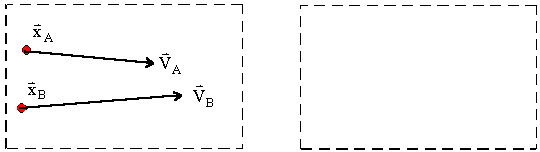In the Lagrangian description of fluid flow, individual fluid particles are "marked," and their positions, velocities, etc. are described as a function of time. In the example shown, particles A and B have been identified. Position vectors and velocity vectors are shown at one instant of time for each of these marked particles. As the particles move in the flow field, their postions and velocities change with time, as seen in the animated diagram. The physical laws, such as Newton's laws and conservation of mass and energy, apply directly to each particle. If there were only a few particles to consider, as in a high school physics experiment with billiard balls, the Lagrangian description would be desirable. However, fluid flow is a continuum phenomenon, at least down to the molecular level. It is not possible to track each "particle" in a complex flow field. Thus, the Lagrangian description is rarely used in fluid mechanics.
• The Eulerian Description is one in which a control volume is defined, within which fluid flow properties of interest are expressed as fields.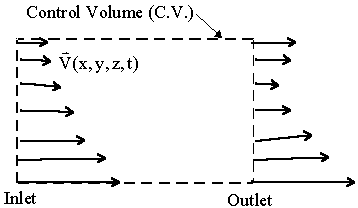In the Eulerian description of fluid flow, individual fluid particles are not identified. Instead, a control volume is defined, as shown in the diagram. Pressure, velocity, acceleration, and all other flow properties are described as fields within the control volume. In other words, each property is expressed as a function of space and time, as shown for the velocity field in the diagram. In the Eulerian description of fluid flow, one is not concerned about the location or velocity of any particular particle, but rather about the velocity, acceleration, etc. of whatever particle happens to be at a particular location of interest at a particular time. Since fluid flow is a continuum phenomenon, at least down to the molecular level, the Eulerian description is usually preferred in fluid mechanics. Note, however, that the physical laws such as Newton's laws and the laws of conservation of mass and energy apply directly to particles in a Lagrangian description. Hence, some translation or reformulation of these laws is required for use with an Eulerian description.
• Example - Pressure field - An example of a fluid flow variable expressed in Eulerian terms is the pressure. Rather than following the pressure of an individual particle, a pressure field is introduced, i.e.
p = p(x,y,z,t).
Note that pressure is a scalar, and is written as a function of space and time (x,y,z, and t). In other words, at a given point in space (x,y, and z), and at some particular time (t), the pressure is defined. In the Eulerian description, it is of no concern which fluid particle is at that location at that time. In fact, whatever fluid particle happens to be at that location at time t experiences the pressure defined above.
• Example - Velocity field - An example of a fluid flow variable expressed in Eulerian terms is the velocity. Rather than following the velocity of an individual particle, a velocity field is introduced, i.e.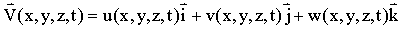Note that since velocity is a vector, it can be split into three components (u,v, and w), all three of which are functions of space and time (x,y,z, and t). In other words, at a given point in space (x,y, and z), and at some particular time (t), the velocity vector is defined. In the Eulerian description, it is of no concern which fluid particle is at that location at that time. In fact, whatever fluid particle happens to be at that location at time t has the velocity defined above.
• Example - Acceleration field - An example of a fluid flow variable expressed in Eulerian terms is the acceleration. Rather than following the acceleration of an individual particle, an acceleration field is introduced, i.e.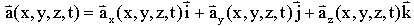.
Note that since acceleration is a vector, it can be split into three components, all three of which are functions of space and time (x,y,z, and t). In other words, at a given point in space (x,y, and z), and at some particular time (t), the acceleration vector is defined. In the Eulerian description, it is of no concern which fluid particle is at that location at that time. In fact, whatever fluid particle happens to be at that location at time t has the acceleration defined above.
• Either description method is valid in fluid mechanics, but the Eulerian description is usually preferred because there are simply too many particles to keep track of in a Lagrangian description.
• The Material Derivative, also called the Total Derivative or Substantial Derivative is useful as a bridge between Lagrangian and Eulerian descriptions.
• Definition of the material derivative - The material derivative of some quantity is simply defined as the rate of change of that quantity following a fluid particle. It is derived for some arbitrary fluid property Q as follows: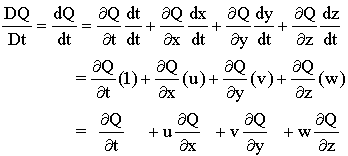In this derivation, dt/dt = 1 by definition, and since a fluid particle is being followed, dx/dt = u, i.e. the x-component of the velocity of the fluid particle. Similarly, dy/dt = v, and dz/dt = w following a fluid particle.
Note that Q can be any fluid property, scalar or vector. For example, Q can be a scalar like the pressure, in which case one gets the material derivative or substantial derivative of the pressure. In other words, dp/dt is the rate of change of pressure following a fluid particle. Or, using the same equations above, Q can be the velocity vector, in which case one gets the material derivative of the velocity, which is defined as the material acceleration, i.e. the rate of change of velocity following a fluid particle.
Note also the notation, DQ/DT, which is used by some authors to emphasize that this is a material or total derivative, as opposed to some partial derivative. DQ/DT is identical to dQ/dt.
The material derivative is a field quantity, i.e. it is expressed in the Eulerian frame of reference as a function of space and time (x,y,z,t). Thus, at some given spatial location (x,y,z) and at some given time (t), DQ/Dt = dQ/dt = the material derivative of Q, and is defined as the total rate of change of Q with respect to time as one follows whatever fluid particle happens to be at that location at that instant of time. Q changes for two reasons: First, if the flow is unsteady, Q changes directly with respect to time. This is called the local or unsteady rate of change of Q. Second, Q changes as the fluid particle migrates or convects to a new location in the flow field. This is called the convective or advective rate of change of Q.
• Example - the material acceleration, following a fluid particle - The material acceleration can be derived as follows: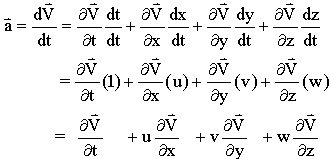Note that dt/dt = 1 by definition, and since a fluid particle is being followed, dx/dt = u, i.e. the x-component of the velocity of the fluid particle. Similarly, dy/dt = v, and dz/dt = w following a fluid particle. The first term on the right hand side is called the local acceleration or the unsteady acceleration. It is only non-zero in an unsteady flow. The last three terms make up the convective acceleration, which is defined as the acceleration due to convection or movement of the fluid particle to a different part of the flow field. The convective acceleration can be non-zero even in a steady flow! In other words, even when the velocity field is not a function of time (i.e. a steady flow), a fluid particle is still accelerated from one location to another.

### Methods of Visualizing Fluid Flows

• A streamline is a line everywhere tangent to the velocity vector at a given instant of time. (A streamline is an instantaneous pattern.) For example, consider simple shear flow between parallel plates. At some instant of time, a streamline can be drawn by connecting the velocity vector lines such that the streamline is everywhere parallel to the local velocity vector. In this example, streamlines are simply horizontal lines.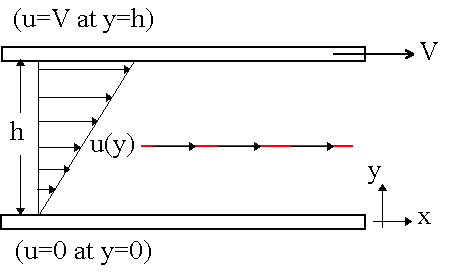• A streakline is the locus of particles which have earlier passed through a prescribed point in space. (A streakline is an integrated pattern.) For example, consider simple shear flow between parallel plates. A streakline is formed by injecting dye into the fluid at a fixed point in space. As time marches on, the streakline gets longer and longer, and represents an integrated history of the dye streak. In this example, streaklines are simply horizontal lines.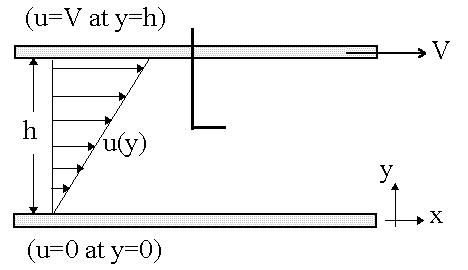• A pathline is the actual path traversed by a given (marked) fluid particle. (A pathline is an integrated pattern.) For example, consider simple shear flow between parallel plates. A pathline is the actual path traversed by a given (marked) fluid particle. A pathline represents an integrated history of where the fluid particle has been. In this example, pathlines are simply horizontal lines.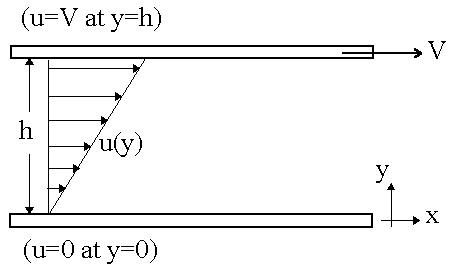• A timeline is a set of fluid particles that form a line segment at a given instant of time. (A timeline is an integrated pattern.) For example, consider simple shear flow between parallel plates. A timeline follows the location of a line of fluid particles. A timeline represents an integrated flow pattern, since the time line continually distorts with time, as shown in the sketch. Notice the no-slip condition in action. The top of the time line moves with the top plate, i.e. at velocity V to the right. The bottom of the timeline, however, stays in the same location at all times, because the bottome plate is not moving.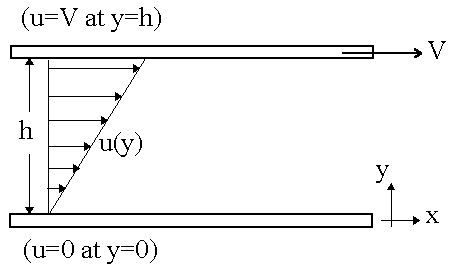• Note: For steady flow, streamlines, streaklines, and pathlines are all identical. However, for unsteady flow, these three flow patterns can be quite different.
전체 0